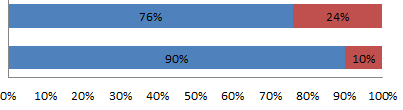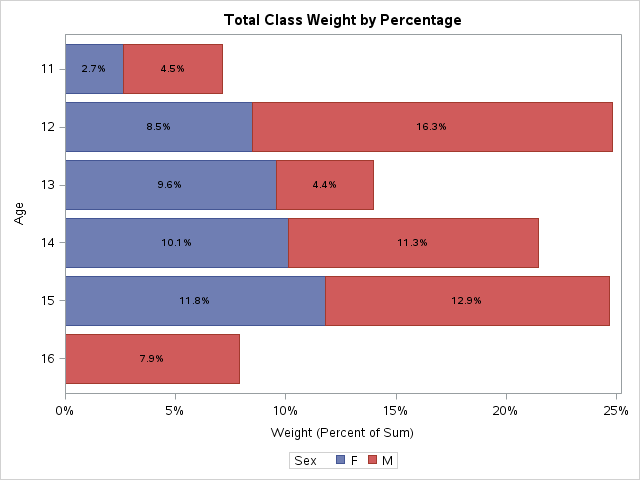DavidPhillips2
Rhodochrosite | Level 12

## Bargraph with an Axis of Percent

Does SAS 9.2 have the ability to display a Bargraph with an Axis of percent?  My goal is to show a percent comparison of variable ‘Status’.  Like the below image.  The code would theoretically look something like this.proc gchart data=enrollment;

hbar enrRepTy /

type=percent

inside=subpct

sumvar=STUDENTS_ENROLLED

pctsum

subgroup=Status

discrete

gaxis=axis1 maxis=axis2 raxis=axis3

legend = legend1;

run; Quit;

1 ACCEPTED SOLUTION

Accepted Solutions

## Re: Bargraph with an Axis of Percent

Has some examples that should get you started.

A common theme has to do with the AXIS definition in the values part and format chosen.

5 REPLIES 5DavidPhillips2
Rhodochrosite | Level 12

## Re: Bargraph with an Axis of Percent

What I could do is make a summary dataset that has the percentages hard coded rather than attempting to calculate the percentage when making the graph.  It is highly likely there is a built in function for this though.

## Re: Bargraph with an Axis of Percent

Can you provide sample data?

## Re: Bargraph with an Axis of Percent

Has some examples that should get you started.

A common theme has to do with the AXIS definition in the values part and format chosen.DavidPhillips2
Rhodochrosite | Level 12

## Re: Bargraph with an Axis of Percent

Ballardw, I found this in one of the examples in your link.  It is straight forward.

data my_data;

input CATEGORY SERIES \$ 3-11 AMOUNT;

datalines;

1 Series A  5.0

2 Series A  6.8

3 Series A  9.2

1 Series B  6.5

2 Series B  6.9

3 Series B  5.6

1 Series C  2.3

2 Series C  3.1

3 Series C  2.3

;

run;

proc sql;

create table my_data as

select *, sum(amount) as bartotal

from my_data

group by category;

quit; run;

data my_data; set my_data;

format catpct percent6.0;

catpct=amount/bartotal;

run;

data my_data; set my_data;

length htmlvar \$500;

htmlvar=

'title='||quote(

'Category: '||trim(left(category))||'0D'x||

'Series: '||trim(left(series))||'0D'x||

'Amount: '||trim(left(amount))||'0D'x||

'Percent: '||trim(left(put(catpct,percent6.0)))||' of '||trim(left(bartotal))

)||

' href="bar6_info.htm"';

run;

proc gchart data=my_data;

hbar category / discrete type=sum sumvar=catpct nostats nolegend

subgroup=series /* this controls the coloring */

autoref clipref cref=graycc space=4.0

maxis=axis1 raxis=axis2

cframe=white coutline=black

html=htmlvar

des='' name="&name";

run;DanH_sas
SAS Super FREQ

## Re: Bargraph with an Axis of Percent

SAS 9.4m2  supports everything you want via PROC SGPLOT (see below). SAS 9.4 supports everything but the segment labels. At SAS 9.2, you will need to pre-summarize as you suggested.

Title "Total Class Weight by Percentage";

proc sgplot data=sashelp.class;

hbar age / response=weight stat=pct group=sex seglabel;

run;Discussion stats
• 5 replies
• 1598 views
• 3 likes
• 4 in conversation